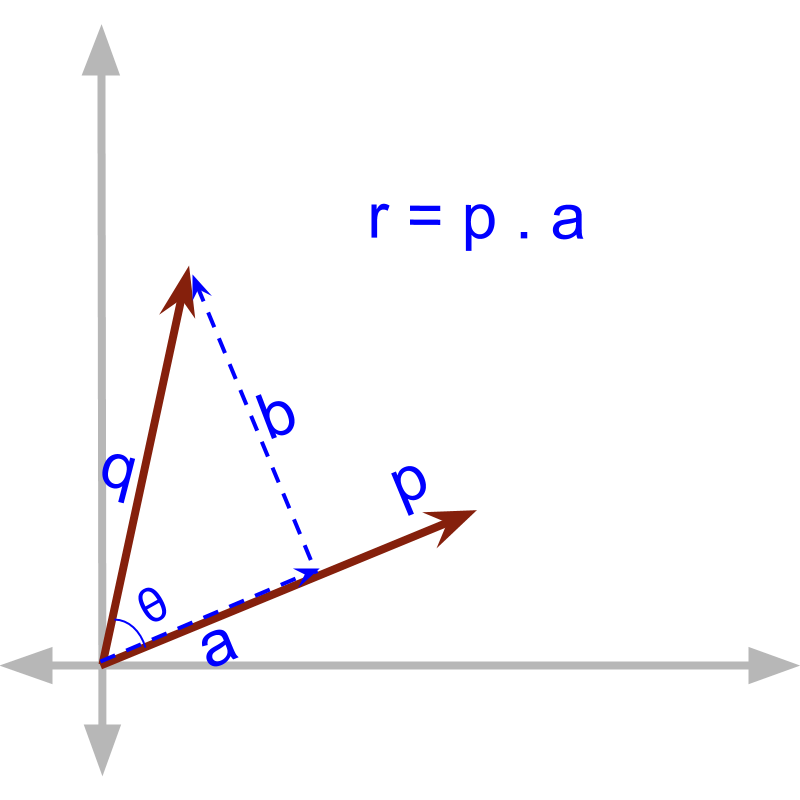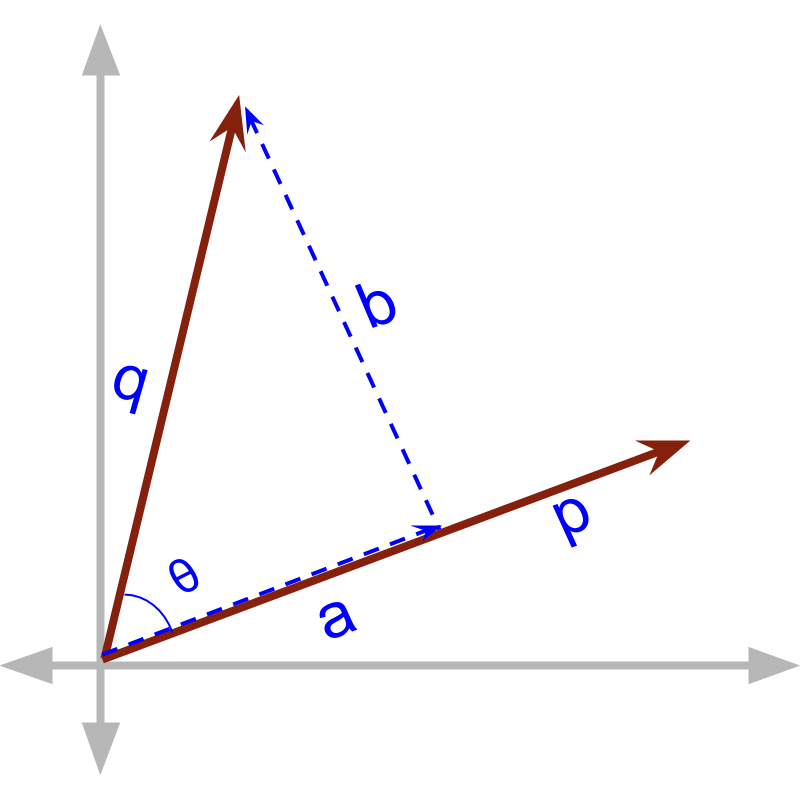maths > vector-algebra

Vector Dot Product : Projection of a vector

what you'll learn...

Overview

Vector Dot Product: Projection form

»  component in parallel is calculated by the angle→  $\stackrel{\to }{p}\cdot \stackrel{\to }{q}$$\vec{p} \cdot \vec{q}$ $=|\stackrel{\to }{p}||\stackrel{\to }{q}|\mathrm{cos}\theta$$= | \vec{p} | | \vec{q} | \cos \theta$
→  Note that $|\stackrel{\to }{a}|=|\stackrel{\to }{q}|\mathrm{cos}\theta$$| \vec{a} | = | \vec{q} | \cos \theta$
→  $\stackrel{\to }{a}$$\vec{a}$ is the projection of $\stackrel{\to }{q}$$\vec{q}$ on $\stackrel{\to }{p}$$\vec{p}$

projectionVector dot product is understood as product between components in parallel to each other.
In finding the component in parallel to one vector the vector is projected on to another. In the figure, $a$$a$ is the projection of $\stackrel{\to }{q}$$\vec{q}$ onto $\stackrel{\to }{p}$$\vec{p}$

That means $a$$a$ can be calculated using vector dot product. That is, the vector dot product can be used to find projection of a vector on a line.Consider the line given by $\stackrel{\to }{s}$$\vec{s}$ and the vector $\stackrel{\to }{p}$$\vec{p}$ as shown in the figure. We know that $\stackrel{\to }{s}\cdot \stackrel{\to }{p}=|\stackrel{\to }{s}||\stackrel{\to }{p}|\mathrm{cos}\theta$$\vec{s} \cdot \vec{p} = | \vec{s} | | \vec{p} | \cos \theta$.
To find the projection of $\stackrel{\to }{p}$$\vec{p}$ on $\stackrel{\to }{s}$$\vec{s}$, the following can be used

$\stackrel{^}{s}\cdot \stackrel{\to }{p}$$\hat{s} \cdot \vec{p}$, where $\stackrel{^}{s}$$\hat{s}$ is unit vector

$\frac{\stackrel{\to }{s}}{|\stackrel{\to }{s}|}\cdot \stackrel{\to }{p}$$\frac{\vec{s}}{| \vec{s} |} \cdot \vec{p}$

Two vectors $\stackrel{\to }{p}$$\vec{p}$ and $\stackrel{\to }{q}$$\vec{q}$ with magnitudes $2$$2$ and $3$$3$ respectively are at an angle ${60}^{\circ }$${60}^{\circ}$. What is the projection of $\stackrel{\to }{p}$$\vec{p}$ on $\stackrel{\to }{q}$$\vec{q}$?

The answer is 'All the above'.

summary

Projection of a vector: Projection of a vector $\stackrel{\to }{p}$$\vec{p}$ on a line in direction $\stackrel{\to }{s}$$\vec{s}$ is
$=\stackrel{\to }{p}\cdot \stackrel{^}{s}$$= \vec{p} \cdot \hat{s}$
where $\stackrel{^}{s}$$\hat{s}$ is the unit vector along $\stackrel{\to }{s}$$\vec{s}$.

Vector dot product can be used to find projection of a vector on a line or on another vector.

Outline